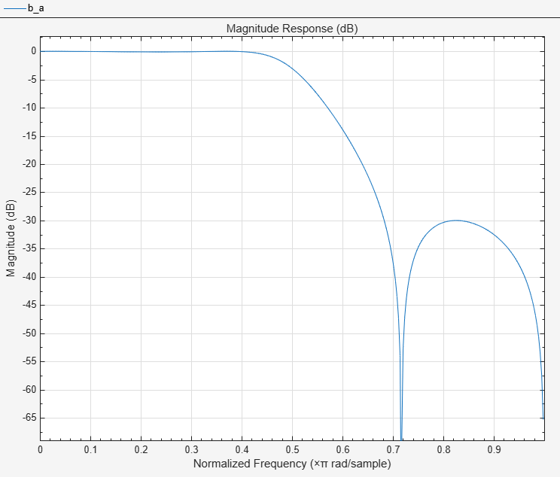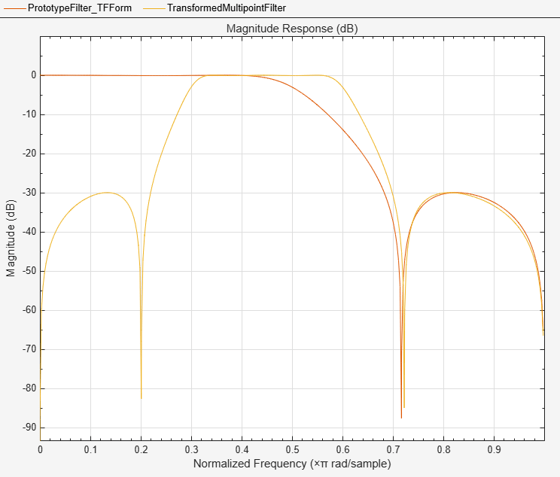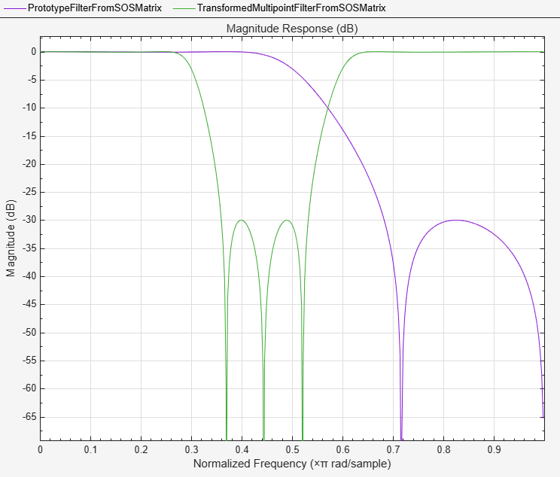# iirlp2xn

Transform IIR lowpass filter to IIR real N-point filter

## Syntax

``````[num,den,allpassNum,allpassDen] = iirlp2xn(b,a,wo,wt)``````
``````[num,den,allpassNum,allpassDen] = iirlp2xn(b,a,wo,wt,pass)``````

## Description

example

``` ```[num,den,allpassNum,allpassDen] = iirlp2xn(b,a,wo,wt)``` transforms an IIR lowpass filter to an IIR real N-point filter.The `iirlp2xn` function returns the numerator and denominator coefficients of the transformed IIR N-point filter. The function also returns the numerator, `allpassNum`, and the denominator, `allpassDen`, of the Nth order allpass mapping filter. The prototype lowpass filter is specified with the numerator `b` and the denominator `a`.The function transforms a real lowpass prototype filter to an N-point filter by applying an Nth-order real lowpass to real multipoint frequency transformation, where N is the number of features being mapped and is given by the length of the vector `wo`. By default, the DC feature is kept at its original location. For more details, see IIR Lowpass to IIR Real N-Point Filter Transformation.```
``` ```[num,den,allpassNum,allpassDen] = iirlp2xn(b,a,wo,wt,pass)``` allows you to specify an additional parameter, `pass` as `'pass'` or `'stop'`, which chooses between using the “Nyquist Mobility” and the “DC Mobility”, respectively. In the case of “Nyquist Mobility”, the DC feature is kept at an original frequency and the Nyquist feature is free to move. In the case of “DC Mobility”, the Nyquist feature stays at its original location and the DC feature is free to move.Frequencies must be normalized to be between 0 and 1, with 1 corresponding to half the sample rate.```

## Examples

collapse all

Transform a lowpass IIR filter to an N-point IIR filter using the `iirlp2xn` function.

Input Lowpass IIR Filter

Design a prototype real IIR lowpass elliptic filter with a gain of about –3 dB at 0.5π rad/sample.

```[b,a] = ellip(3,0.1,30,0.409); fvtool(b,a)```Transform Filter Using `iirlp2xn`

Transform the real prototype lowpass filter to an IIR real N-point filter with one passband.

Specify the prototype filter as a vector of numerator and denominator coefficients, `b` and `a` respectively.

`[num,den] = iirlp2xn(b,a,[-0.5 0.5],[0.3 0.6],"pass");`

Compare the magnitude response of the filters using FVTool.

```hvft = fvtool(b,a,num,den); legend(hvft,"Prototype Filter (TF Form)",... "Transformed Multipoint Filter");```You can also specify the input lowpass IIR filter as a matrix of coefficients. Pass the second-order section matrices as inputs. The transformed filter is a fourth-order section filter with one stopband. The numerator and the denominator coefficients of the transformed filter are given by `num2` and `den2`, respectively.

```ss = tf2sos(b,a); [num2, den2] = iirlp2xn(ss(:,1:3),ss(:,4:6),[-0.5 0.5],... [0.3 0.6],"stop");```

Compare the magnitude response of the filters using FVTool.

To visualize the magnitude response of the fourth-order section filter, first pass the filter coefficients to the `dsp.FourthOrderSectionFilter` object. Then, use this object as an input to the FVTool.

```fos = dsp.FourthOrderSectionFilter(Numerator=num2,... Denominator=den2); hvft = fvtool(ss,fos); legend(hvft,"Prototype Filter (SOS Form)",... "Transformed Multipoint Filter")```## Input Arguments

collapse all

Numerator coefficients of the prototype lowpass IIR filter, specified as either:

• Row vector –– Specifies the values of [b0, b1, …, bn], given this transfer function form:

`$H\left(z\right)=\frac{B\left(z\right)}{A\left(z\right)}=\frac{{b}_{0}+{b}_{1}{z}^{-1}+\cdots +{b}_{n}{z}^{-n}}{{a}_{0}+{a}_{1}{z}^{-1}+\cdots +{a}_{n}{z}^{-n}},$`

where n is the order of the filter.

• Matrix –– Specifies the numerator coefficients in the form of an P-by-(Q+1) matrix, where P is the number of filter sections and Q is the order of each filter section. If Q = 2, the filter is a second-order section filter. For higher-order sections, make Q > 2.

`$b=\left[\begin{array}{ccccc}{b}_{01}& {b}_{11}& {b}_{21}& ...& {b}_{Q1}\\ {b}_{02}& {b}_{12}& {b}_{22}& ...& {b}_{Q2}\\ ⋮& ⋮& ⋮& \ddots & ⋮\\ {b}_{0P}& {b}_{1P}& {b}_{2P}& \cdots & {b}_{QP}\end{array}\right]$`

In the transfer function form, the numerator coefficient matrix bik of the IIR filter can be represented using the following equation:

`$H\left(z\right)=\prod _{k=1}^{P}{H}_{k}\left(z\right)=\prod _{k=1}^{P}\frac{{b}_{0k}+{b}_{1k}{z}^{-1}+{b}_{2k}{z}^{-2}+\cdots +{b}_{Qk}{z}^{-Q}}{{a}_{0k}+{a}_{1k}{z}^{-1}+{a}_{2k}{z}^{-2}+\cdots +{a}_{Qk}{z}^{-Q}},$`

where,

• a –– Denominator coefficients matrix. For more information on how to specify this matrix, see `a`.

• k –– Row index.

• i –– Column index.

When specified in the matrix form, b and a matrices must have the same number of rows (filter sections) Q.

Data Types: `single` | `double`
Complex Number Support: Yes

Denominator coefficients for a prototype lowpass IIR filter, specified as one of these options:

• Row vector –– Specifies the values of [a0, a1, …, an], given this transfer function form:

`$H\left(z\right)=\frac{B\left(z\right)}{A\left(z\right)}=\frac{{b}_{0}+{b}_{1}{z}^{-1}+\cdots +{b}_{n}{z}^{-n}}{{a}_{0}+{a}_{1}{z}^{-1}+\cdots +{a}_{n}{z}^{-n}},$`

where n is the order of the filter.

• Matrix –– Specifies the denominator coefficients in the form of an P-by-(Q+1) matrix, where P is the number of filter sections and Q is the order of each filter section. If Q = 2, the filter is a second-order section filter. For higher-order sections, make Q > 2.

`$a=\left[\begin{array}{ccccc}{a}_{01}& {a}_{11}& {a}_{21}& \cdots & {a}_{Q1}\\ {a}_{02}& {a}_{12}& {a}_{22}& \cdots & {a}_{Q2}\\ ⋮& ⋮& ⋮& \ddots & ⋮\\ {a}_{0P}& {a}_{1P}& {a}_{2P}& \cdots & {a}_{QP}\end{array}\right]$`

In the transfer function form, the denominator coefficient matrix aik of the IIR filter can be represented using the following equation:

`$H\left(z\right)=\prod _{k=1}^{P}{H}_{k}\left(z\right)=\prod _{k=1}^{P}\frac{{b}_{0k}+{b}_{1k}{z}^{-1}+{b}_{2k}{z}^{-2}+\cdots +{b}_{Qk}{z}^{-Q}}{{a}_{0k}+{a}_{1k}{z}^{-1}+{a}_{2k}{z}^{-2}+\cdots +{a}_{Qk}{z}^{-Q}},$`

where,

• b –– Numerator coefficients matrix. For more information on how to specify this matrix, see `b`.

• k –– Row index.

• i –– Column index.

When specified in the matrix form, a and b matrices must have the same number of rows (filter sections) P.

Data Types: `single` | `double`
Complex Number Support: Yes

Frequency values to transform from the prototype filter, specified as a row vector. Frequencies in `wo` should be normalized to be between `0` and `1`, with `1` corresponding to half the sample rate.

The value of N equals the length of the `wo` vector. Length of vectors `wo` and `wt` must be the same.

Data Types: `single` | `double`

Desired frequency locations in the transformed target filter, specified as a row vector. Frequencies in `wt` should be normalized to be between `0` and `1`, with `1` corresponding to half the sample rate.

Length of vectors `wo` and `wt` must be the same.

Data Types: `single` | `double`

Choice of passband or stopband at DC, specified as either:

• `"pass"` –– “Nyquist Mobility”. The DC feature is kept at an original frequency and the Nyquist feature is free to move.

• `"stop"` –– “DC Mobility”. The Nyquist feature stays at its original location and the DC feature is free to move.

## Output Arguments

collapse all

Numerator coefficients of the transformed multipoint filter, returned as one of the following:

• Row vector of length Nn+1, where n is the order of the input filter and N is the number of features being mapped. The value of N equals the length of the `wo` vector.

The `num` output is a row vector when the input coefficients `b` and `a` are row vectors.

• P-by-(QN+1) matrix, where P is the number of filter sections, Q is the order of each section of the transformed filter, and N is the number of features being mapped and is given by the length of the vector `wo`.

The `num` output is a matrix when the input coefficients `b` and `a` are matrices.

Data Types: `single` | `double`
Complex Number Support: Yes

Denominator coefficients of the transformed multipoint filter, returned as one of the following:

• Row vector of length Nn+1, where n is the order of the input filter and N is the number of features being mapped. The value of N equals the length of the `wo` vector.

The `den` output is a row vector when the input coefficients `b` and `a` are row vectors.

• P-by-(QN+1) matrix, where P is the number of filter sections, Q is the order of each section of the transformed filter, and N is the number of features being mapped and is given by the length of the vector `wo`.

The `den` output is a matrix when the input coefficients `b` and `a` are matrices.

Data Types: `single` | `double`
Complex Number Support: Yes

Numerator coefficients of the mapping filter, returned as a row vector.

Data Types: `single` | `double`

Denominator coefficients of the mapping filter, returned as a row vector.

Data Types: `single` | `double`

collapse all

### IIR Lowpass to IIR Real N-Point Filter Transformation

IIR Lowpass to IIR Real N-Point Filter transformation effectively places N features of the original filter, located at frequencies `wo1`, … ,`woN`, at the required target frequency locations, `wt1`, … ,`wtM`. The function `iirlp2xn` requires that N and M are equal.

Relative positions of other features of the original filter are the same in the target filter for the Nyquist mobility and are reversed in DC mobility. For the Nyquist mobility this means that it is possible to select two features of an original filter, F1 and F2, with F1 preceding F2. Feature F1 will still precede F2 after the transformation. However, the distance between F1 and F2 will not be the same before and after the transformation. For DC mobility feature F2 will precede F1 after the transformation.

Choice of the feature subject to this transformation is not restricted to the cutoff frequency of an original lowpass filter. In general it is possible to select any feature; e.g., the stopband edge, the DC, the deep minimum in the stopband, or other ones. Select features such that there is no band overlap when creating N bands around the unit circle.

IIR lowpass to IIR real N-point filter transformation can also be used to transforming other types of filters, for example, notch filters or resonators can be easily replicated at a number of required frequency locations. A good application would be an adaptive tone cancellation circuit reacting to the changing number and location of tones.

 Krukowski, A., G.D. Cain, and I. Kale. “Custom Designed High-Order Frequency Transformations for IIR Filters.” In 38th Midwest Symposium on Circuits and Systems. Proceedings, 1:588–91. Rio de Janeiro, Brazil: IEEE, 1996.

 Cain, G.D., A. Krukowski and I. Kale, “High Order Transformations for Flexible IIR Filter Design,” VII European Signal Processing Conference (EUSIPCO'94), vol. 3, pp. 1582-1585, Edinburgh, United Kingdom, September 1994.